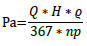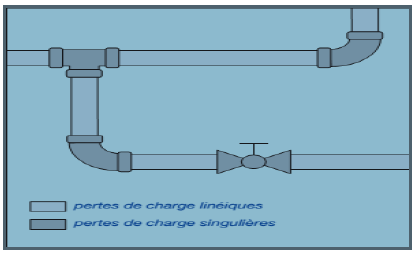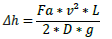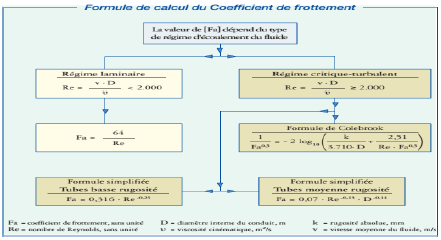E) The power P:
The power absorbed by the fluid is expressed as a function of the manometric height, The flow rate and the efficiency of the pump according to the following relation:F) Pressure losses:
These are pressure losses due to the resistance of the fluid in
movement. They may be linear or singular:
– the linear losses appear along the lines;
– the singular losses are manifested in special parts
Alter the direction or cross-section of the fluid (eg reductions,
Derivatives, connections, junctions, valves, filters, etc.).Figure 16: Types of Load Loss

Calculation of linear pressure losses
For each meter of tube, the linear head losses can be calculated with
formula:With:
Δ𝐡 = unitary linear pressure drop, Par; V = average liquid velocity, m / s
Fa = coefficient of friction, without unit; D = inner diameter of tube, m

When we know the diameter of the tube, the velocity of the liquid and its density, the only
The parameter to be determined remains the friction coefficient: coefficient which varies according to:
Calculation of linear pressure losses

For each meter of tube, the linear head losses can be calculated with

formula:With: Δ𝐡 = unitary linear pressure drop, Par; V = average liquid velocity, m / s Fa = coefficient of friction, without unit; D = inner diameter of tube, m When we know the diameter of the tube, the velocity of the liquid and its density, the only The parameter to be determined remains the friction coefficient: coefficient which varies according to: Equation 13: Number of Reynolds Where: Re = Reynolds number, without unit V = average fluid velocity, m / s D = inner diameter of tube, m Υ = kinematic viscosity of the fluid, m2 / s Depending on this number, the movement of the fluid can be considered as: – laminar if Re is less than 2,000 – critical if Re is between 2,000 and 2,500 – turbulent if Re is greater than 2,500Figure 17: Summary table of calculation of coefficient of friction

Calculation of singular pressure drops
These pressure drops are caused by special parts that alter the direction or cross-section
Of fluid passage. They can be calculated using one of the following methods:
the method of equivalent lengths, it replaces each special part by a
Linear tube segment capable of generating the same pressure drops.
– the direct method, it uses coefficients that vary with the shape and dimensions
Special parts.
– nominal flow method, it uses, for each special part, the value of its sound
Nominal flow: that is to say the flow rate which corresponds to a loss of unit pressure
Predefined (for example 1 bar).
For our study, we will use the equivalent length method.